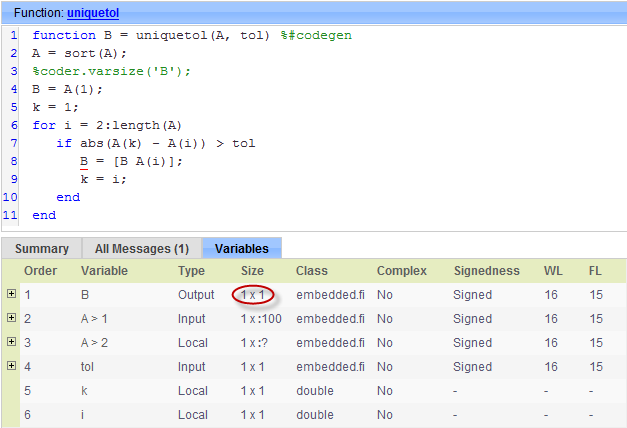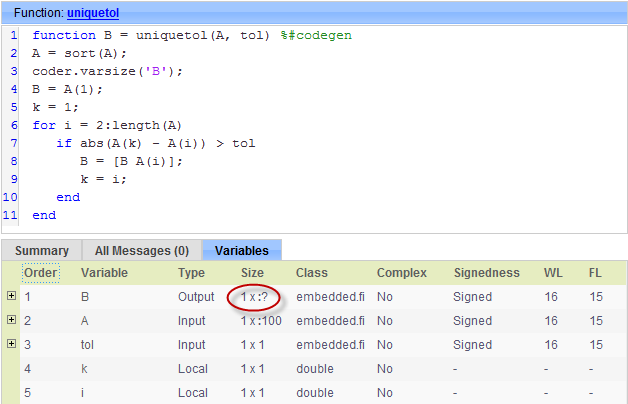## Accelerate Code for Variable-Size Data

Variable-size data is data whose size might change at run time. MATLAB® supports bounded and unbounded variable-size data for code generation. Bounded variable-size data has fixed upper bounds. This data can be allocated statically on the stack or dynamically on the heap. Unbounded variable-size data does not have fixed upper bounds. This data must be allocated on the heap. By default, for MEX and C/C++ code generation, support for variable-size data is enabled and dynamic memory allocation is enabled for variable-size arrays whose size exceeds a configurable threshold.

### Disable Support for Variable-Size Data

By default, for MEX and C/C++ code acceleration, support for variable-size data is enabled. You modify variable sizing settings at the command line.

1. Create a configuration object for code generation.

`cfg = coder.mexconfig;`

2. Set the `EnableVariableSizing` option:

`cfg.EnableVariableSizing = false;`

3. Using the `-config` option, pass the configuration object to `fiaccel` :

```fiaccel -config cfg foo ```

### Control Dynamic Memory Allocation

By default, dynamic memory allocation is enabled for variable-size arrays whose size exceeds a configurable threshold. If you disable support for variable-size data, you also disable dynamic memory allocation. You can modify dynamic memory allocation settings at the command line.

1. Create a configuration object for code acceleration. For example, for a MEX function:

`mexcfg = coder.mexconfig;`

2. Set the `EnableDynamicMemoryAllocation` option:

SettingAction
`mexcfg.EnableDynamicMemoryAllocation=false;`
Dynamic memory allocation is disabled. All variable-size data is allocated statically on the stack.
`mexcfg.EnableDynamicMemoryAllocation=true;`
Dynamic memory allocation is enabled for all variable-size arrays whose size (in bytes) is greater than or equal to the value specified using the `Dynamic memory allocation threshold` parameter. Variable-size arrays whose size is less than this threshold are allocated on the stack.

3. Optionally, if you set `Enable dynamic memory allocation` to `true`, configure ```Dynamic memory allocation threshold``` to fine tune memory allocation.

4. Using the `-config` option, pass the configuration object to `fiaccel`:

```fiaccel -config mexcfg foo ```

### Accelerate Code for MATLAB Functions with Variable-Size Data

Here is a basic workflow that generates MEX code.

1. In the MATLAB Editor, add the compilation directive `%#codegen` at the top of your function.

This directive:

• Indicates that you intend to generate code for the MATLAB algorithm

• Turns on checking in the MATLAB Code Analyzer to detect potential errors during code generation

2. Address issues detected by the Code Analyzer.

In some cases, the MATLAB Code Analyzer warns you when your code assigns data a fixed size but later grows the data, such as by assignment or concatenation in a loop. If that data is supposed to vary in size at run time, you can ignore these warnings.

3. Generate a MEX function using `fiaccel`. Use the following command-line options:

• `-args {coder.typeof...}` if you have variable-size inputs

• `-report` to generate a code generation report

For example:

```fiaccel -report foo -args {coder.typeof(0,[2 4],1)} ```
This command uses `coder.typeof` to specify one variable-size input for function `foo`. The first argument, `0`, indicates the input data type (`double`) and complexity (`real`). The second argument, `[2 4]`, indicates the size, a matrix with two dimensions. The third argument, `1`, indicates that the input is variable sized. The upper bound is 2 for the first dimension and 4 for the second dimension.

Note

During compilation, `fiaccel` detects variables and structure fields that change size after you define them, and reports these occurrences as errors. In addition, `fiaccel` performs a runtime check to generate errors when data exceeds upper bounds.

4. Fix size mismatch errors:

You try to change the size of data after its size has been locked.Declare the data to be variable sized.See Diagnosing and Fixing Size Mismatch Errors.
5. Fix upper bounds errors

MATLAB cannot determine or compute the upper boundSpecify an upper bound.See Specify Upper Bounds for Variable-Size Arrays and Diagnosing and Fixing Size Mismatch Errors.
MATLAB attempts to compute an upper bound for unbounded variable-size data.If the data is unbounded, enable dynamic memory allocation. See Control Dynamic Memory Allocation
6. Generate C/C++ code using the `fiaccel` function.

### Accelerate Code for a MATLAB Function That Expands a Vector in a Loop

#### About the MATLAB Function uniquetol

This example uses the function `uniquetol`. This function returns in vector `B` a version of input vector `A`, where the elements are unique to within tolerance `tol` of each other. In vector `B`, `abs`(`B`(`i`) - `B`(`j`)) > `tol` for all `i` and `j`. Initially, assume input vector `A` can store up to 100 elements.

```function B = uniquetol(A, tol) A = sort(A); B = A(1); k = 1; for i = 2:length(A) if abs(A(k) - A(i)) > tol B = [B A(i)]; k = i; end end```

#### Step 1: Add Compilation Directive for Code Generation

Add the `%#codegen` compilation directive at the top of the function:

```function B = uniquetol(A, tol) %#codegen A = sort(A); B = A(1); k = 1; for i = 2:length(A) if abs(A(k) - A(i)) > tol B = [B A(i)]; k = i; end end```

#### Step 2: Address Issues Detected by the Code Analyzer

The Code Analyzer detects that variable `B` might change size in the `for-` loop. It issues this warning:

```The variable 'B' appears to change size on every loop iteration. Consider preallocating for speed.```

In this function, vector `B` should expand in size as it adds values from vector `A`. Therefore, you can ignore this warning.

#### Step 3: Generate MEX Code

To generate MEX code, use the `fiaccel` function.

1. Generate a MEX function for `uniquetol`:

```T = numerictype(1, 16, 15); fiaccel -report uniquetol -args {coder.typeof(fi(0,T),[1 100],1),coder.typeof(fi(0,T))} ```

Executing this command generates a compiler error:

```??? Size mismatch (size [1 x 1] ~= size [1 x 2]). The size to the left is the size of the left-hand side of the assignment. ```
2. Open the error report and select the Variables tab.The error indicates a size mismatch between the left-hand side and right-hand side of the assignment statement `B = [B A(i)];`. The assignment ```B = A(1)``` establishes the size of `B` as a fixed-size scalar (1 x 1). Therefore, the concatenation of `[B A(i)]` creates a 1 x 2 vector.

#### Step 4: Fix the Size Mismatch Error

To fix this error, declare `B` to be a variable-size vector.

1. Add this statement to the `uniquetol` function:

`coder.varsize('B');`

It should appear before `B` is used (read). For example:

```function B = uniquetol(A, tol) %#codegen A = sort(A); coder.varsize('B'); B = A(1); k = 1; for i = 2:length(A) if abs(A(k) - A(i)) > tol B = [B A(i)]; k = i; end end```

The function `coder.varsize` declares every instance of `B` in `uniquetol` to be variable sized.

2. Generate code again using the same command:

`fiaccel -report uniquetol -args {coder.typeof(fi(0,T),[1 100],1),coder.typeof(fi(0,T))}`

In the current folder, `fiaccel` generates a MEX function for `uniquetol` named `uniquetol_mex` and provides a link to the code generation report.

3. Click the View report link.

4. In the code generation report, select the Variables tab.The size of variable `B` is `1x:?`, indicating that it is variable size with no upper bounds.

#### Step 5: Compare Execution Speed of MEX Function to Original Code

Run the original MATLAB algorithm and MEX function with the same inputs for the same number of loop iterations and compare their execution speeds.

1. Create inputs of the correct class, complexity, and size to pass to the `uniquetol` MATLAB and MEX functions.

```x = fi(rand(1,90), T); tol = fi(0, T); ```
2. Run the original `uniquetol` function in a loop and time how long it takes to execute 10 iterations of the loop.

```tic; for k=1:10, b = uniquetol(x,tol); end; tSim=toc ```
3. Run the generated MEX function with the same inputs for the same number of loop iterations.

```tic; for k=1:10, b = uniquetol_mex(x,tol); end; tSim_mex=toc ```
4. Compare the execution times.

```r = tSim/tSim_mex ```

This example shows that generating a MEX function using `fiaccel` greatly accelerates the execution of the fixed-point algorithm.